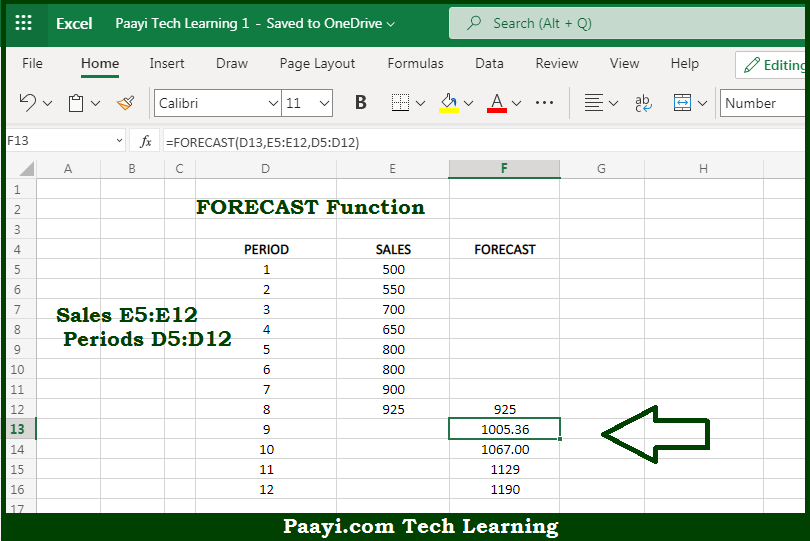# Learn How to Use Microsoft Excel FORECAST Function

Written by | 0 Comments | 585 Views

In this article, you will learn how to use the Microsoft Excel FORECAST function and its prime function in Microsoft Excel. You will also get to know the Microsoft Excel FORECAST function return value and syntax with the help of some examples.

Microsoft Excel FORECAST Function

The main purpose of the Microsoft Excel FORECAST function is to predict the value along with the linear trend. That implies, with the help of the FORECAST function you can able to predict a value based on already given values along with a linear trend. The FORECAST function calculates future value predictions using linear regression and can be used to predict numeric values like sales, expenses, inventory, measurements, etc. So, with the help of the FORECAST function, you can able to predict the value along with the linear trend.

Return Value of FORECAST Function

The return value will be the predicted value.

Syntax of FORECAST Function

=FORECAST(x, known-ys, known-xs)

Where the arguments:

• x: This is the x value data point to use for the prediction.
• known-yx: This is the dependent array or range of data (y values).
• known-xs: This is the dependent array or range of data (x values).

## How to Use Microsoft Excel FORECAST Function?So we know that Microsoft Excel FORECAST function you can able to predict the value along with the linear trend. That implies, with the help of the FORECAST function you can able to predict a value based on already given values along with a linear trend. The FORECAST function calculates future value predictions using linear regression and can be used to predict numeric values like sales, expenses, inventory, measurements, etc. So, with the help of the FORECAST function, you can able to predict the value along with the linear trend.